# Class 8 Maths NCERT Solutions for Chapter – 6 Squares and Square Roots Ex – 6.3

## Squares and Square Roots

Question 1.
What could be the possible ‘one’s’ digits of the square root of each of the following numbers?
(i)
9801
(ii) 99856
(iii) 998001
(iv) 657666025

Solution:
The possible ‘one’s’ digits of the square root of the numbers :
(i) 9801 is 1 or 9
(ii) 99856 is 4 or 6
(iii) 998001 is 1 or 9
(iv) 657666025 is 5

Question 2.
Without doing any calculation, find the numbers which are surely not perfect squares :
(i)
153
(ii) 257
(iii) 408
(iv) 441

Solution:
We know that a number ending with 2, 3, 7 or 8 is never a perfect square.
Therefore,
(i) 153 is not a perfect square.
(ii) 257 is not a perfect square.
(iii) 408 is not a perfect square.
(iv) 441 may be a perfect square.
So, 153, 257 and 408 are surely not perfect squares.

Question 3.
Find the square roots of 100 and 169 hy the method of repeated subtraction.

Solution:
From 100, we subtract successive odd numbers starting from 1 as under: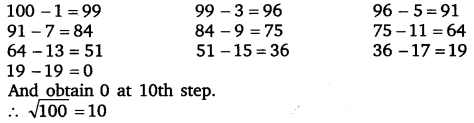From 169, we subtract successive odd numbers starting from 1 as under:Question 4.
Find the square roots of the following numbers by the Prime Factorisation Method :
(i)
729
(ii) 400
(iii) 1764
(iv) 4096
(v) 7744
(vi) 9604
(vii) 6929
(viii) 9216
(ix) 529
(x) 8100

Solution: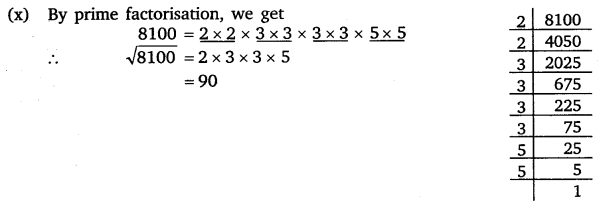Question 5.
For each of the following numbers, find the smallest whole number by which it should be multiplied so as to get a perfect square number. Also find the square root of the square number so obtained.
(i)
252
(ii) 180
(iii) 1008
(iv) 2028
(v) 1458
(vi) 768

Solution: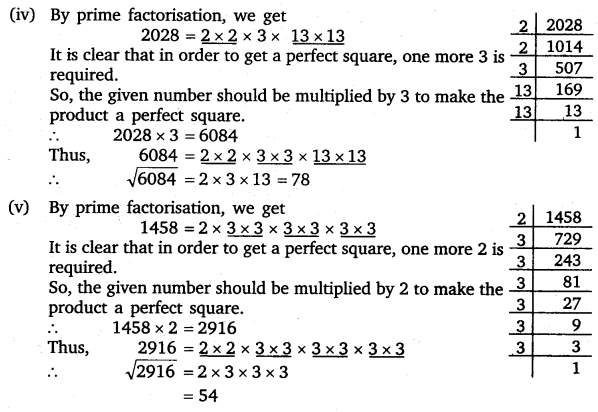Question 6.
For each of the following numbers. find the smallest whole number by which it should be divided so as to get a perfect square. Also find the square root of the square number so obtained.
(i)
252
(ii) 2925
(iii) 396
(iv) 2645
(v) 2800
(vi) 1620

Solution: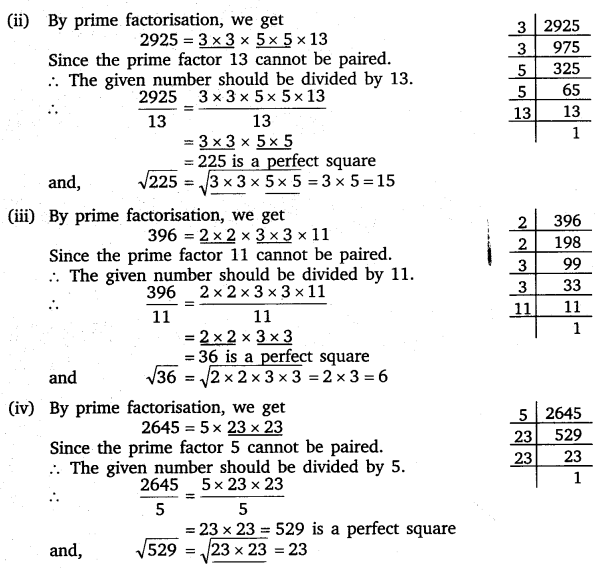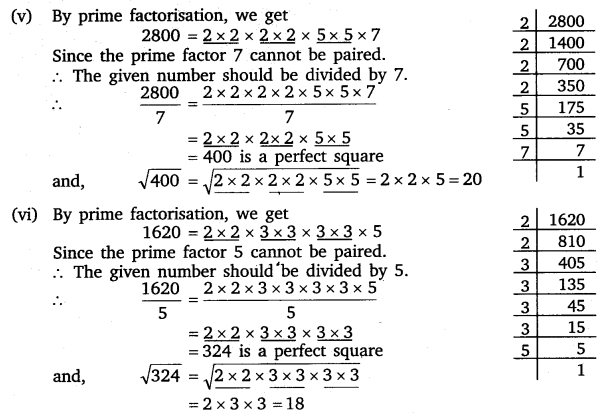Question 7.
The students of Class VIII of a school donated ? 2401 in all, for Prime Minister’s National Relief Fund. Each student donated as many rupees as the number of students in the class. Find the number of students in the class.

Solution:
Let x be the number of students in the class. Therefore, total money donated by students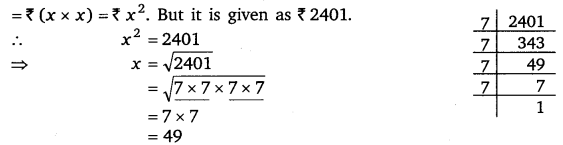Hence, the number of the students in the class is 49.

Question 8.
2025 plants are to be planted in a garden in such a way that each row contains as many plants as the number of rows. Find the number of rows and the number of plants in each row.

Solution:
let x be the number of rows and x be the number of plants in each row.
∴ The total number of plants = x x x = x2
But is it given as 2025.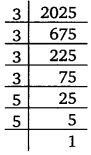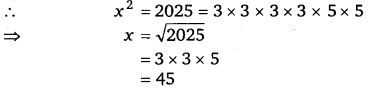Hence, the number of rows is 45 and the number of plants in each row is also 45.

Question 9.
Find the smallest square number that is divisible by each of the numbers 4, 9 and 10.

Solution:
The smallest number divisible by each one of the numbers 4, 9 and 10 is their LCM., which is (2 x 2 x 9 x 5), i.e., 180.
Now, 180 = 2 x 2 x 3 x 3 x 5
To make it a perfect square, it must be multiplied by 5.∴ Required number = 180 x 5 = 900

Question 10.
Find the smallest square number that is divisible by each of the numbers 8, 15 and 20.

Solution:
The smallest number divisible by each of the numbers 8, 15 and 20 is their LCM., which is
(2 x 2 x 5 x 2 x 3), i.e., 120.
Now, 120 = 2 x 2 x 2 x 3 x 5
To make it a perfect square, it must be multiplied by
2 x 3 x 5, i.e., 30.∴ Required number = 120 x 30 = 3600

#### Class 8 Maths NCERT Solutions for Chapter – 6 Squares and Square Roots Ex – 6.4

Lorem ipsum dolor sit amet, consectetur adipiscing elit. Phasellus cursus rutrum est nec suscipit. Ut et ultrices nisi. Vivamus id nisl ligula. Nulla sed iaculis ipsum.

Company Name